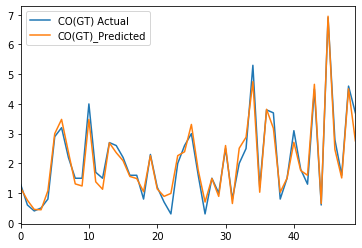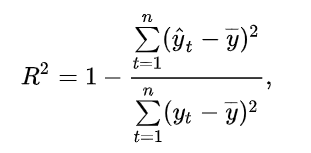# Tutorial: Linear Regression – Sklearn regression model (#3/281120191333)

In the previous part, we tried to build a model by trying to explain the level of carbon monoxide pollution based on temperature and pressure. Despite the use of shift, the model proved to be inefficient. We will return to this model later. Meanwhile, we will now perform a linear regression model in Sklearn and Tensorflow.

In :
```import pandas as pd
```
Out:
Unnamed: 0 Unnamed: 0.1 Unnamed: 0.1.1 Date Time CO(GT) PT08.S1(CO) C6H6(GT) PT08.S2(NMHC) NOx(GT) PT08.S4(NO2) PT08.S5(O3) T RH AH DATE Month Weekday Weekday_name Hours
0 0 0 0 10/03/2004 18.00.00 2.6 1360.0 11.9 1046.0 166.0 1692.0 1268.0 13.6 48.9 0.7578 2004-03-10 18:00:00 3 2 Wednesday 18
1 1 1 1 10/03/2004 19.00.00 2.0 1292.0 9.4 955.0 103.0 1559.0 972.0 13.3 47.7 0.7255 2004-03-10 19:00:00 3 2 Wednesday 19
2 2 2 2 10/03/2004 20.00.00 2.2 1402.0 9.0 939.0 131.0 1555.0 1074.0 11.9 54.0 0.7502 2004-03-10 20:00:00 3 2 Wednesday 20

3 rows × 22 columns

In :
```del df['Unnamed: 0']
```

## Checking the correlation with the result variable

We will consider carbon monoxide CO pollution as the result variable

In :
```CORREL = df.corr().sort_values('CO(GT)')
PCK = CORREL['CO(GT)'].to_frame().sort_values('CO(GT)')
PCK
```
Out:
CO(GT)
PT08.S3(NOx) -0.715683
Weekday -0.140231
RH 0.020122
AH 0.025227
T 0.025639
Unnamed: 0.1 0.042099
Unnamed: 0.1.1 0.042099
Month 0.112291
Hours 0.344071
PT08.S4(NO2) 0.631854
NO2(GT) 0.682774
NOx(GT) 0.773677
PT08.S5(O3) 0.858762
PT08.S1(CO) 0.886114
PT08.S2(NMHC) 0.918386
C6H6(GT) 0.932584
CO(GT) 1.000000
In :
```import matplotlib.pyplot as plt

plt.figure(figsize=(10,8))
PCK.plot(kind='barh', color='#6d9eeb')
plt.title('Correlation with the resulting variable: CO ', fontsize=20)
plt.xlabel('Correlation level')
plt.ylabel('Continuous independent variables')
```
Out:
`Text(0, 0.5, 'Continuous independent variables')`

## Part one: Linear regression in Sklearn

Declares X, y variables into the model. To the set of describing variables does not give data in text and date format.

In :
```df.columns
```
Out:
```Index(['Unnamed: 0.1', 'Unnamed: 0.1.1', 'Date', 'Time', 'CO(GT)',
'PT08.S1(CO)', 'C6H6(GT)', 'PT08.S2(NMHC)', 'NOx(GT)', 'PT08.S3(NOx)',
'NO2(GT)', 'PT08.S4(NO2)', 'PT08.S5(O3)', 'T', 'RH', 'AH', 'DATE',
'Month', 'Weekday', 'Weekday_name', 'Hours'],
dtype='object')```
In :
```X = df[['PT08.S1(CO)','C6H6(GT)','PT08.S2(NMHC)','NOx(GT)','PT08.S3(NOx)','NO2(GT)','PT08.S4(NO2)','PT08.S5(O3)','T','RH', 'AH'
,'Month','Weekday','Hours']].values
y = df['CO(GT)'].values
```

I divide the collection into training variables and test variables.

In :
```from sklearn.model_selection import train_test_split
from sklearn.linear_model import LinearRegression

X_train, X_test, y_train, y_test = train_test_split(X, y, test_size=0.2, random_state=0)
```

I am building a regression model.

In :
```regressor = LinearRegression()
regressor.fit(X_train, y_train)
```
Out:
`LinearRegression(copy_X=True, fit_intercept=True, n_jobs=None, normalize=False)`
In :
```import numpy as np

y_pred = regressor.predict(X_test)
y_pred = np.round(y_pred, decimals=2)
```

Comparison of variables from the model with real variables.

In :
```dfKK = pd.DataFrame({'CO(GT) Actual': y_test, 'CO(GT)_Predicted': y_pred})
```
Out:
CO(GT) Actual CO(GT)_Predicted
0 1.3 1.18
1 0.6 0.76
2 0.4 0.45
3 0.5 0.44
4 0.8 1.08
In :
```dfKK.to_csv('c:/TF/AAr2.csv')
```
In :
```from sklearn import metrics

```
Out:
`<matplotlib.axes._subplots.AxesSubplot at 0x215167771d0>`In :
```print('Mean Squared Error:     ', metrics.r2_score(y_test, y_pred))
```
```Mean Squared Error:      0.9202224478702138
```

The R Square parameter shows that the model has close to perfection fit into empirical variables.

## How to calculate the R Square parameter?

In the SKlearn package, the R-Square parameter is calculated easily and pleasantly. This is not always the case, for example in the TensorFlow library the calculation of this indicator is difficult. Therefore, we will now calculate the R-Square index on foot.

It is a statistic used in the context of statistical models whose main purpose is either the prediction of future outcomes or the testing of hypotheses, on the basis of other related information. It provides a measure of how well observed outcomes are replicated by the model, based on the proportion of total variation of outcomes explained by the model.We will now calculate this difficult formula step by step for our example of linear regression.

### Point 1. We calculate the square error

The quadratic error is the difference between the actual value and the estimation. We put this difference squared.</span>

In :
```dfKK.head(5)
```
Out:
CO(GT) Actual CO(GT)_Predicted
0 1.3 1.18
1 0.6 0.76
2 0.4 0.45
3 0.5 0.44
4 0.8 1.08
In :
```dfKK['SSE'] = (dfKK['CO(GT) Actual'] - dfKK['CO(GT)_Predicted'])**2
```
Out:
CO(GT) Actual CO(GT)_Predicted SSE
0 1.3 1.18 0.0144
1 0.6 0.76 0.0256
2 0.4 0.45 0.0025

### Point 2. We calculate the average empirical value of y

In :
```dfKK['ave_y'] = dfKK['CO(GT) Actual'].mean()
```
Out:
CO(GT) Actual CO(GT)_Predicted SSE ave_y
0 1.3 1.18 0.0144 2.086004
1 0.6 0.76 0.0256 2.086004
2 0.4 0.45 0.0025 2.086004

### Point 3. We calculate the difference between empirical values y and the average of empirical values y

In :
```dfKK['SST'] = (dfKK['CO(GT) Actual'] - dfKK['ave_y'])**2
```
Out:
CO(GT) Actual CO(GT)_Predicted SSE ave_y SST
0 1.3 1.18 0.0144 2.086004 0.617803
1 0.6 0.76 0.0256 2.086004 2.208209
2 0.4 0.45 0.0025 2.086004 2.842610

### Point 4. We calculate the difference between sum of SST and sum of SSE¶

In :
```Sum_SST = dfKK['SST'].sum()
print('Sum_SST :',Sum_SST)
Sum_SSE = dfKK['SSE'].sum()
print('Sum_SSE :',Sum_SSE)
SSR = Sum_SST - Sum_SSE
```
```Sum_SST : 3829.9533119658117
Sum_SSE : 305.5443
```

### Point 5. We calculate the R Square parameter

In :
```r2 = SSR/Sum_SST
print('R Square parameter: ',r2)
```
```R Square parameter:  0.9202224478702138
```